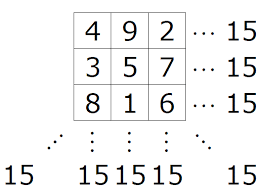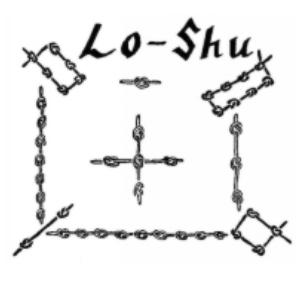Math Puzzles

# Puzzle time! Can you solve these magic square questions?

2.2k views

 1 Introduction 2 But why are they called magic? 3 How do you solve a magic square? 4 Applications of magic square 5 Conclusion 6 About Cuemath 7 FAQs 8 External references

12 November  2020

## Introduction

### What is a magic square in math?

A magic square is a square array of numbers consisting of both positive and negative numbers which are arranged in such a way that the sum of the numbers in any horizontal, vertical, or main diagonal line is always the same number.In this above figure, you can see that each row, column and main diagonal add up to 15.

Also, try:

## But why are they called magic?

It appears to be that from old times they were associated with the heavenly and mysterious world. The soonest record of magic squares is from China in around 2200 BC also it is designated to "Lo-Shu". A legend says that the Emperor Yu saw this magic square on the back of a divine turtle in the Yellow River.The dark knots show even numbers and the white knots show odd numbers. Magic squares were first referenced in the Western world in the work of Theon of Smyrna. They were additionally utilized by Arab astrologers in the ninth century to help work out horoscopes.

The work by the Greek mathematician Moschopoulos in 1300 A.D. helped to spread information about magic squares. So here we are presently, over 700 years after the fact, and teachers are utilizing them in class for critical thinking and practising addition.

You can make similar magic squares of order 3 using different numbers. Can you try analysing patterns in the numbers that work?

## How do you solve a magic square?

These are simple problems where simple addition and subtraction skills are used to solve these magic square puzzles.

Magic square solving methods is shown in the below video:

## Tricks to solve magic square:

Trick to solve 3*3 magic square:

Trick to solve 4*4 magic square:

Solve these sheets to develop your problem-solving skills and reasoning:

## Applications of magic square

• Music: The main area of the application of magic squares to music is in rhythm, rather than notes.This is important to music as it shows the size of the magic square, which is how many pulses or sub-divisions there are in the sequence, this will indicate how and where to apply it.
• Soduku: Sudoku was first introduced in 1979 and became popular in Japan during the 1980’s (Pegg Weisstein, 2006). It has recently become a very popular puzzle in Europe, but it is actually a form of Latin square.Nowadays, these games are available in playstore and most of them play to learn and improve their mental skills.

## Conclusion

Did you successfully solve the magic squares?

Successful or Unsuccesful. You definitely had a good learning experience.

Why is it important to solve magic square puzzles?

Mathematicians today do not need to speculate and attach meaning to magic squares to make them important, as has been done in the past with Chinese and other myths. The squares were thought to be mysterious and magic, although now it is clear that they are just ways of arranging numbers and symbols using certain rules.

• In Magic Squares, you can use natural numbers starting from 1.
• In Magic Squares, you can have fractions.
• In Magic Squares, you can have repetitions.
• In Magic Squares, you can have irrationals.

There is no boundary!

Magic Square tells us there are no shortcuts to answer, you have to do the calculations to reach there. And it's not the answer, but the method is what is more important!

Did you realize the importance of attempting such math puzzles?

Math puzzles enable children to visualize concepts and thus understand math concepts better and faster. In a way, math puzzles remove the tag of 'difficulty and boredom' from maths and make it fun and exciting even for the children who do not like math.

Cuemath, a student-friendly mathematics platform, conducts regular Online Live Classes for academics and skill-development and their Mental Math App, on both iOS and Android, is a one-stop solution for kids to develop multiple skills.Understand the  Cuemath fee structure and sign up for a free trial.

## What is a magic square?

A magic square is any n x n array of numbers where each of the n2 elements appears only once. Also the sum of the elements in each row, column, and diagonal have the same value.

## How do you do a magic square?

Solving an Odd-Numbered Magic Square
sum = 3 * [(9 + 1) / 2]
sum = 3 * (10 / 2)
sum = 3 * (5)
sum = 15.
The magic constant for a 3x3 square is 15.
All rows, columns, and diagonals must add up to this number.

## How do you make a 3x3 magic square?

In a magic square you have to add 3 numbers again and again. Therefore the average sum of three numbers is 45:3=15. The number 15 is called the magic number of the 3x3 square. You can also achieve 15, if you add the middle number 5 three times.

## What is Ramanujan magic square?

A magic square is an NxN matrix in which every row, column, and diagonal add up to the same number. Srinivasa Ramanujan was an Indian mathematician. ... Ramanujan created a super magic square. The top row is his birthdate (December 22, 1887).